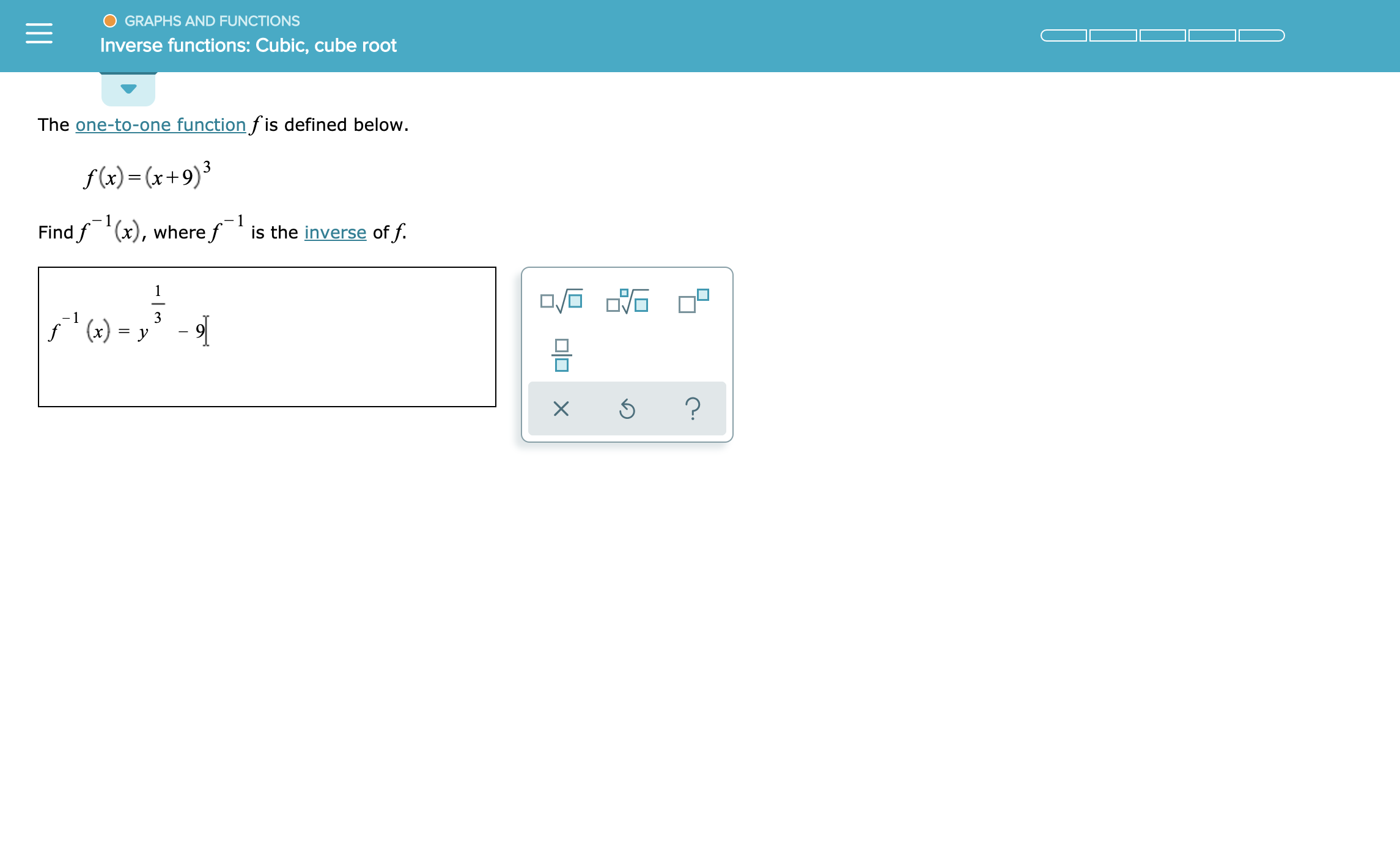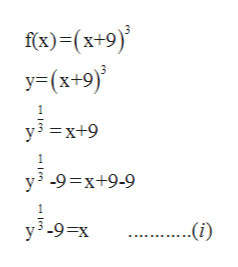GRAPHS AND FUNCTIONSInverse functions: Cubic,cube rootThe one-to-one function fis defined below.f (x)x+9)31is the inverse of f.Find f (x), wheref1()y3?X

Question

see attachmenthelp_outlineImage TranscriptioncloseGRAPHS AND FUNCTIONS Inverse functions: Cubic,cube root The one-to-one function fis defined below. f (x)x+9)3 1 is the inverse of f. Find f (x), wheref 1 ()y 3 ? X fullscreen
Step 1

The function f(x) = (x+9)3. Find f-1(x)?

Step 2

To find inverse of the given function, find ...help_outlineImage Transcriptionclosefx)-(x+9) y(x+9) y3 =x+9 y3 -9 x+9-9 y3-9-x ...(i) en fullscreen

Want to see the full answer?

See Solution

Want to see this answer and more?

Our solutions are written by experts, many with advanced degrees, and available 24/7

See Solution
Tagged in

Calculus